# PHP Date and Time

This article was written and published to give a succinct explanation of how PHP handles dates and times with an example. To handle date and time in your PHP application, I'll demonstrate how to use some of the most popular and well-known PHP functions in this post. So let's begin now without further ado.

## PHP date() function

To format a given date and time, use PHP's date() function. It accepts a format string and an optional timestamp as its two parameters. The timestamp specifies the date and time to format, whereas the format string specifies the desired output format. The current date and time serve as the timestamp's default value. As an illustration.

```<?php
\$cdt = date("Y-m-d H:i:s");
echo "Current date and time: <b>", \$cdt, "</b>";
?>```

The following snapshot shows the sample output that this PHP code produces.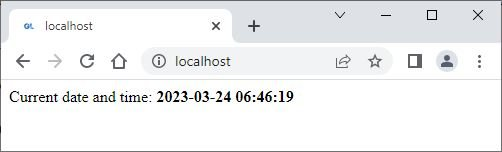The format string passed to the date() function in this example is "Y-m-d H:i:s." This format string specifies that the date and time be formatted as follows:

Format Code Meaning
Y The full year in 4 digits (e.g. 2023)
- A separator character
m The month as a number with leading zeros (e.g. 03 for March)
d The day of the month as a number with leading zeros (e.g. 24)
A space character
H The hour in 24-hour format with leading zeros (e.g. 10 for 10am)
: A separator character
i The minute with leading zeros (e.g. 30)
s The second with leading zeros (e.g. 00)

## PHP strtotime() function

PHP's strtotime() function converts a string representation of a date and time to a Unix timestamp. It can parse a variety of date and time formats and return a timestamp value representing the number of seconds since January 1, 1970, at 00:00:00 UTC. As an example:

```<?php
\$dateString = "March 24, 2023";
\$timeStamp = strtotime(\$dateString);
echo "Total number of seconds since January 1, 1970: <b>", \$timeStamp, "</b>";
?>```

This PHP code produces an output that will be similar to the following snapshot. However, I chose March 24, 2023. Based on the date, the number of seconds since January 1, 1970, will be altered.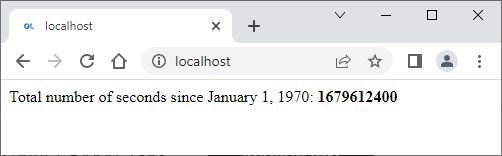This number of seconds value represented in the above snapshot represents the seconds that have elapsed since January 1, 1970, at 00:00:00 UTC, until March 24, 2023, at 00:00:00.

## PHP time() function

PHP's time() function returns the current Unix timestamp, which represents the number of seconds since January 1, 1970, at 00:00:00 UTC. As an example:

```<?php
\$currentTime = time();
echo "Total number of seconds since January 1, 1970: <b>", \$currentTime, "</b>";
?>```

The current date is March 24, 2023, and the current time is 11:34:30. As a result, the output of this PHP code when executed on my local server is shown in the snapshot below: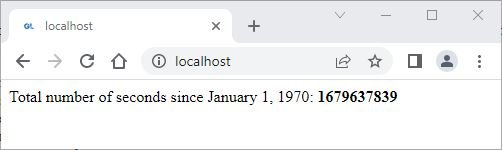This value represents the current time in seconds since January 1, 1970.

## PHP mktime() function

The mktime() function generates a Unix timestamp based on a set of parameters such as the hour, minute, second, month, day, and year. Here's an illustration:

PHP Code
```<?php
\$timestamp = mktime(0, 0, 0, 3, 24, 2023);
echo \$timestamp;
?>```
Output
`1679612400`

This is the Unix timestamp for March 24, 2023 at midnight.

## PHP date_create() function

The date create() function makes a new DateTime object, which can be used to do different things with dates and times. As an example:

PHP Code
```<?php
\$dateString = '2023-03-24 11:44:00';
\$date = date_create(\$dateString);
echo date_format(\$date, 'Y-m-d H:i:s');
?>```
Output
`2023-03-24 11:44:00`

## PHP date_diff() function

The date_diff() function is used to calculate the difference between two dates and times, and return the result as a DateInterval object. As an example:

PHP Code
```<?php
\$date1 = date_create('2022-06-04');
\$date2 = date_create('2023-03-24');
\$diff = date_diff(\$date1, \$date2);

echo \$diff->format('%a days');
?>```
Output
`293 days`

This PHP code computes the time difference between two dates and displays the result in terms of days. The following is a breakdown of what each line of code does:

• The first line creates a new DateTime object called \$date1 using the date_create() function. The date and time of this object is set to June 4, 2022, which is specified as a string in the format 'YYYY-MM-DD'.
• The second line creates a new DateTime object called \$date2 using the date_create() function. The date and time of this object is set to March 24, 2023, which is also specified as a string in the format 'YYYY-MM-DD'.
• The third line calculates the difference between \$date1 and \$date2 using the date_diff() function. This function returns a DateInterval object representing the difference between the two dates.
• The fourth line formats the difference between the two dates as a string using the format() method of the DateInterval object. In this case, the %a specifier is used to display the difference in terms of the number of days between the two dates. The resulting string is then printed to the screen using the echo statement.

## PHP strftime() function

In PHP, the strftime() function is used to format a timestamp into a string based on the user's locale settings. This function requires two inputs: a format string and a timestamp.

The format string is similar to the date() function's format string, but it also supports locale-specific formatting options. For example, in the user's preferred language, you can use %A to display the full weekday name or %B to display the full month name. As an example:

```<?php
setlocale(LC_TIME, 'en_US.utf8');
\$timestamp = time();

\$dateString = strftime('%A, %B %d, %Y', \$timestamp);
echo "Date: <b>", \$dateString, "</b>";
?>```

The sample output is shown in the snapshot below.This PHP code formats the current date and time as a string using the strftime() function and displays it in HTML using the echo statement. Here's a breakdown of what each line of code does:

• The first line sets the locale to US English using the setlocale() function. This tells PHP to use the appropriate language and formatting rules for dates and times in the US.
• The second line employs the time() function to obtain the current timestamp. This function returns the number of seconds that have passed since the Unix epoch (January 1, 1970 at 00:00:00 UTC).
• The third line uses the strftime() function to convert the timestamp to a string. The format string %A, %B %d, and %Y is used to display, separated by commas and spaces, the weekday name, month name, day of the month, and year. The string that results is saved in the variable \$dateString.
• The fourth line uses the echo statement to output an HTML string to the screen. The string includes the label "Date:" in bold text, followed by the formatted date and time string stored in the variable \$dateString.

## PHP date and time examples

Here's an example of how to use PHP to print the current/present date.

```<?php
echo "Today's Date is: <b>" . date('d/m/Y') . "</b>";
?>```

Here is the sample output.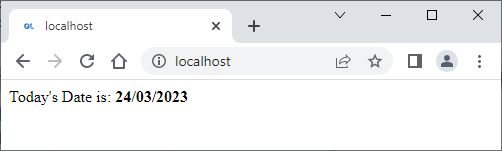Here is an example showing how to print the name of the current day using PHP.

```<?php
\$DayName = date("l");
echo "Today is " . \$DayName;
?>```

Here is an example output from the above PHP example for printing the day's name:Here's an example of how to print the current date and month name in PHP.

```<?php
echo "Current Date: " . date("d M, Y");
?>```

The following is an example output generated by the above example code to print the current date with the month name in PHP: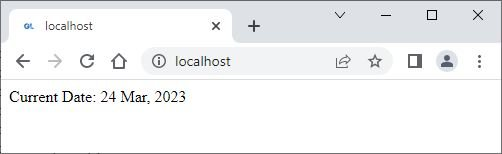The preceding PHP code can also be written as

```<?php
\$DateDay = date("d");
\$DateMonthName = date("M");
\$DateYear = date("Y");

echo "Current Date: " . \$DateDay . " " . \$DateMonthName . ", " . \$DateYear;
?>```

The following is the last example of this post that prints the current time along with AM and PM.

PHP Code
```<?php
echo "The Current Time is: <b>" . date("h:i:s a") . "</b>";
?>
```
Output
`The Current Time is: 07:39:24 am`

However, depending on the current time, you will get a different result in your case.

PHP Online Test

« Previous Tutorial Next Tutorial »